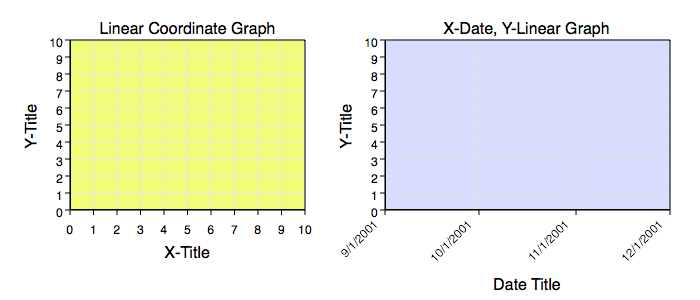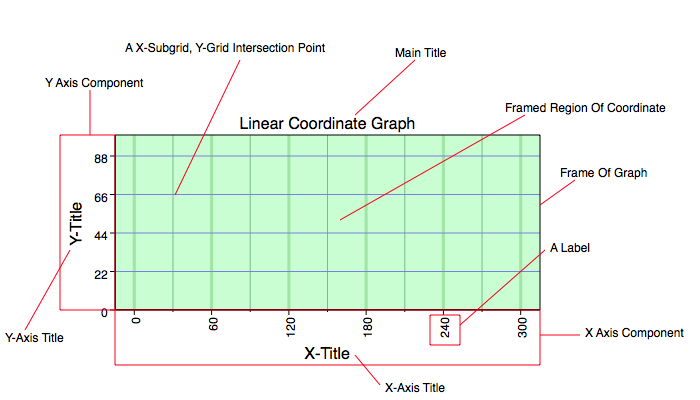Graph IDEGraphs ► Single Coordinate Graph

A Single Coordinate Graph is the usual type of graph which displays only one coordinate. The coordinate can be of any type, linear, log, angular, radial, nonuniform and mixed or non-mixed amongst those types. The coordinate can also have non-orthogonal dimensions. However, there needs to be only one coordinate per point on the graph; contrast that to the Multiple Coordinate Graph.

The figure below shows two examples of a single coordinate graph without any data on them.The figure below annotates the major components of the graph:Note the x-axis and how the tick placement does not have to coincide with the left and right sides of the frame of the graph. The ticks can float along the graph by varying the tick x-minimum or the graph's x-minimum for example.

Inspector Editor

The Inspector Editor for the Single Coordinate Graph is described below.Automatically Reset Fields And Graph

Set Using Auto Scaler : Selecting this button autoscales the graph according to the settings in the Auto Scaler inspector.

Indigenous Coordinate Limits

This specifies the actual limits of the coordinate system represented by the graph and those limits are defined at the graph frame. When you change these limits then you should also probably change the tick discretization limits to the same values.

X-Minimum : The x-minimum value of the graph.

X-Maximum : The x-maximum value of the graph.

Y-Minimum : The y-minimum value of the graph.

Y-Maximum : The y-maximum value of the graph.

Tick Discretization

This specifies the tick locations along the axes defined in the coordinate shown by the axes. Note that the ticks "float" which is to say that they don't follow the Indigenous Coordinate Limits.

X-Minimum : The x-minimum tick value of the graph. Usually this is the same as the x-minimum value of the graph.

X-Maximum : The x-maximum tick value of the graph. Usually this is the same as the x-maximum value of the graph.

Y-Minimum : The y-minimum tick value of the graph. Usually this is the same as the y-minimum value of the graph.

Y-Maximum : The y-maximum tick value of the graph. Usually this is the same as the y-maximum value of the graph.

Apply Values Shown above

Apply : Updates the graph to correspond to the values shown above the button. Note that entering a value in a text field will not change the graph even if the return key is clicked. That is because all of the numbers are related and updating the graph based upon only one of the values can produce a inconsistent state.

Coordinate Mappings

Graphics embedded on the graph are in reference to the Indigenous Coordinate Limits and will appear to translate and scale according to those limits relative to the page view coordinate system. However, those graphics do not actually map, only the mapping relative to the page view changes. However, there is also another mapping type which is the coordinate mapping and is determine by a function, normally a linear function. This mapping maps the actual data relative to the graph coordinate (not the page view coordinate). The data remains the same however and is not mapped.

x = mx + b : This is the function of the mapping. Click this button to change that function to include a absolute value of x.

m : The slope of the linear mapping.

b : The y-intercept of the linear mapping.

This mapping feature makes more sense for polar and logarithmic graphs where the x-values need to be mapped from degrees to radians for polar coordinates and in the case of logarithmic graphs the mapping function includes base, power and reference coefficients. In the case of logarithmic graphs the base coefficient also effects the base of the graph labels while in scientific notation. So, the mapping can be a simple function, can be a unit designation or can be a label formatting requirement.

Reference Frame

This specifies the reference frame location of the graph. Note that the reference frame is the frame that coincides with the Indigenous Coordinate Limits location but in graphic view coordinate.

Left Edge : The left edge of the graph in units and coordinate of the Graphic View.

Bottom Edge : The bottom edge of the graph in units and coordinate of the Graphic View.

Width : The width of the graph in units and coordinate of the Graphic View.

Height : The height of the graph in units and coordinate of the Graphic View.

© Copyright 1993-2019 by VVimaging, Inc. (VVI); All Rights Reserved. Please email support@vvi.com with any comments you have concerning this documentation. See Legal for trademark and legal information.